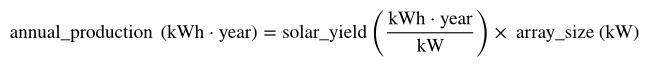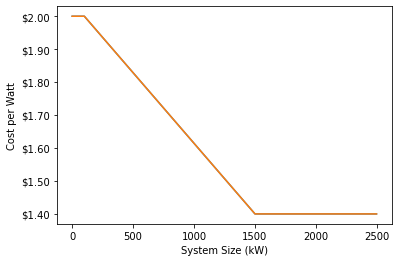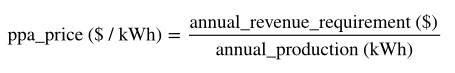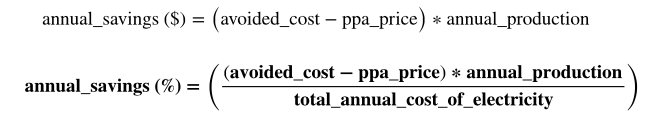Station A Knowledge Base

# How does Station A calculate the financials for a solar system?

## The financials of a solar system are represented as typical savings, or the percentage utility bill reduction we expect, net of any payments to the solar provider.

The financials of a solar system are represented as typical savings, or the percentage utility bill reduction we expect, net of any payments to the solar provider.

To get to this value, we calculate the technical solar potential or solar system size, as described above, along with the following two other crucial intermediate numbers (with more detail behind these calculations below):

1. Solar yield: The expected energy production from a typical solar installation at this location, over a year
2. PPA price: The expected market price for a Power Purchase Agreement (PPA)

### Step 1: Compute the Solar yield

In order to figure out the sizing and savings potential of a solar installation, we need to determine the solar yield, the number of kWh we expect the system to produce annually for each kW of capacity installed. We do this by looking for the nearest US Weather Station and using TMY3 weather data as the weather in a typical year. TMY3 weather years are created by taking the actual weather from a different year for each month, choosing the year in the period 1991-2010 that has the median average temperature in that month.

We then use NREL's PVWatts to calculate the solar yield for an installation at this building, using the following assumptions:

 Input Assumed Value Array Capacity 500 kW Azimuth 180° DC:AC Ratio 1.2 Efficiency 96.0% System Losses 7.8% Tilt 11°

### Step 2: Compute the Annual Production

Once we have the solar yield, we can multiply the system size by solar yield to get the expected annual production for that solar array:### Step 3: Compute the PPA Price

We calculate the PPA price based on the expected return for the developer. In order to do this, we calculate the cost to the developer of building the solar installation (the "CAPEX"), use that to determine how much revenue the installation must generate in a typical year to meet the developers Internal Rate of Return ("IRR") hurdle, and then back into the PPA price that would allow the developer to collect that much revenue. To give more detail on each of those steps:

#### a. Calculate the CAPEX

We calculate the CAPEX based on the size of the project and the cost per watt to build. We determine cost per watt based on the system size, with the relationship shown in this graph:#### b. Account for the Investment Tax Credit

We assume that all solar installations are subsidized by the Investment Tax Credit ("ITC"). The ITC provides a tax credit worth 26% of the cost of a solar project, and we assume it is fully monetized.

#### c. Assume an Annual Revenue Requirement

We assume a 10% requirement for IRR and require that the project generates revenue equal to 10% of the CAPEX in a typical year.

#### d. Calculate the PPA Price

We then use the solar yield to determine the typical annual production for the solar array. We use that to conclude:### Step 4: Compute the Bill Savings

#### a. Estimate the Avoided Cost

To understand the customer's savings, we have to know how much money they save by not consuming electricity from the grid, which is known as their "avoided cost." Replacing a kWh from the grid with a kWh from their rooftop solar array affects the different types of utility charges differently:

 Charge Type Portion Avoided by Solar Energy Charges 100% Demand Charges 30% Fixed Charges 0%

We use these assumptions and our data on the cost of electricity to calculate the avoided cost for each kWh of solar energy produced.

#### b. Put it all together

Given the values above, we calculate the projects typical annual savings as: